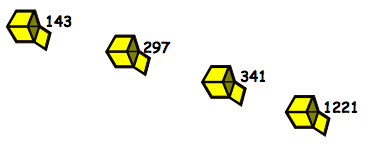#### You may also like### Consecutive Numbers

An investigation involving adding and subtracting sets of consecutive numbers. Lots to find out, lots to explore.### Roll These Dice

Roll two red dice and a green dice. Add the two numbers on the red dice and take away the number on the green. What are all the different possible answers?### Domino Square

Use the 'double-3 down' dominoes to make a square so that each side has eight dots.

# What's in the Box?

##### Age 7 to 11Challenge Level

Four numbers in little boxes are put into a special big box that does a multiplication, then four new numbers come out at the end:We only used whole numbers to go in, so, what multiplication might have gone on in the big box to get the answers in the picture above?

What was the largest number that could have been used to multiply by, in that big box?

Imagine four new boxes now (with new numbers in) and the large box multiplying by a different number this time. The numbers that come out are these:What would be the number that the big box is multiplying by?
How are you working these out?
Discuss with others and see if there are different ways that you found the answers.